# Integral manifold

(diff) ← Older revision | Latest revision (diff) | Newer revision → (diff)

A set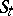of points of the phase space (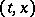-space) of the system(*)

filled by the integral curves of this system (cf. Integral curve), defined for alland forming a manifold in-space. The dimension of the section ofby the plane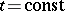is usually called the dimension of the integral manifold. In the definition of an integral manifold, the requirement that it be a manifold is sometimes replaced by the requirement that the setbe representable analytically by an equation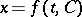with a functiondefined for allinand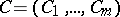in some domainand possessing a specific smoothness in,for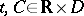. The integral manifold is then called-dimensional and of the same smoothness as the function.

Examples. An integral curve of a periodic solution of the system (*), that is, a periodic integral curve; the family of integral curves of the system (*) formed by a family of quasi-periodic solutions of (*), filling an-dimensional torus in the-space when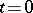, that is, an-dimensional toroidal integral manifold; etc.

The integral manifolds that have been most extensively studied are the toroidal manifolds, that is, setsthat are tori for any fixed. These manifolds are widely encountered in systems of type (*) describing oscillatory processes.

How to Cite This Entry:
Integral manifold. Encyclopedia of Mathematics. URL: http://encyclopediaofmath.org/index.php?title=Integral_manifold&oldid=17392
This article was adapted from an original article by A.M. Samoilenko (originator), which appeared in Encyclopedia of Mathematics - ISBN 1402006098. See original article